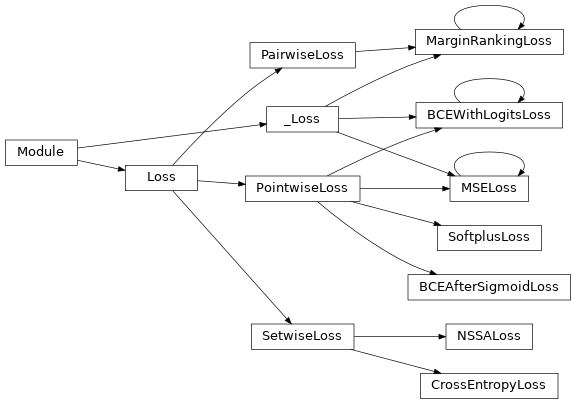# Loss Functions¶

Loss functions integrated in PyKEEN.

## Functions¶

 `get_loss_cls`(query) Get the loss class.

## Classes¶

 `BCEAfterSigmoidLoss`([reduction]) A loss function which uses the numerically unstable version of explicit Sigmoid + BCE. `SoftplusLoss`([reduction]) A loss function for the softplus. `NSSALoss`([margin, adversarial_temperature, …]) An implementation of the self-adversarial negative sampling loss function proposed by [sun2019]. `CrossEntropyLoss`([reduction]) Evaluate cross entropy after softmax output. `MarginRankingLoss`([margin, size_average, …]) A wrapper around the PyTorch margin ranking loss. `MSELoss`([size_average, reduce, reduction]) A wrapper around the PyTorch mean square error loss. `BCEWithLogitsLoss`([weight, size_average, …]) A wrapper around the numeric stable version of the PyTorch binary cross entropy loss.

## Class Inheritance Diagram¶